# [Python人工智能] 三十八.Keras构建无监督学习Autoencoder模型及MNIST聚类可视化详解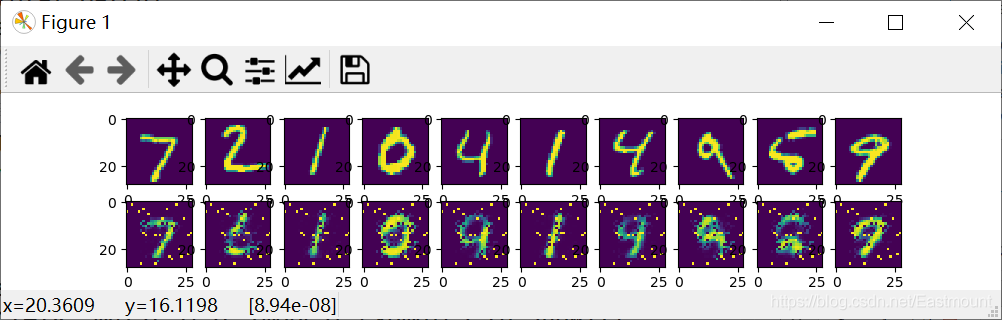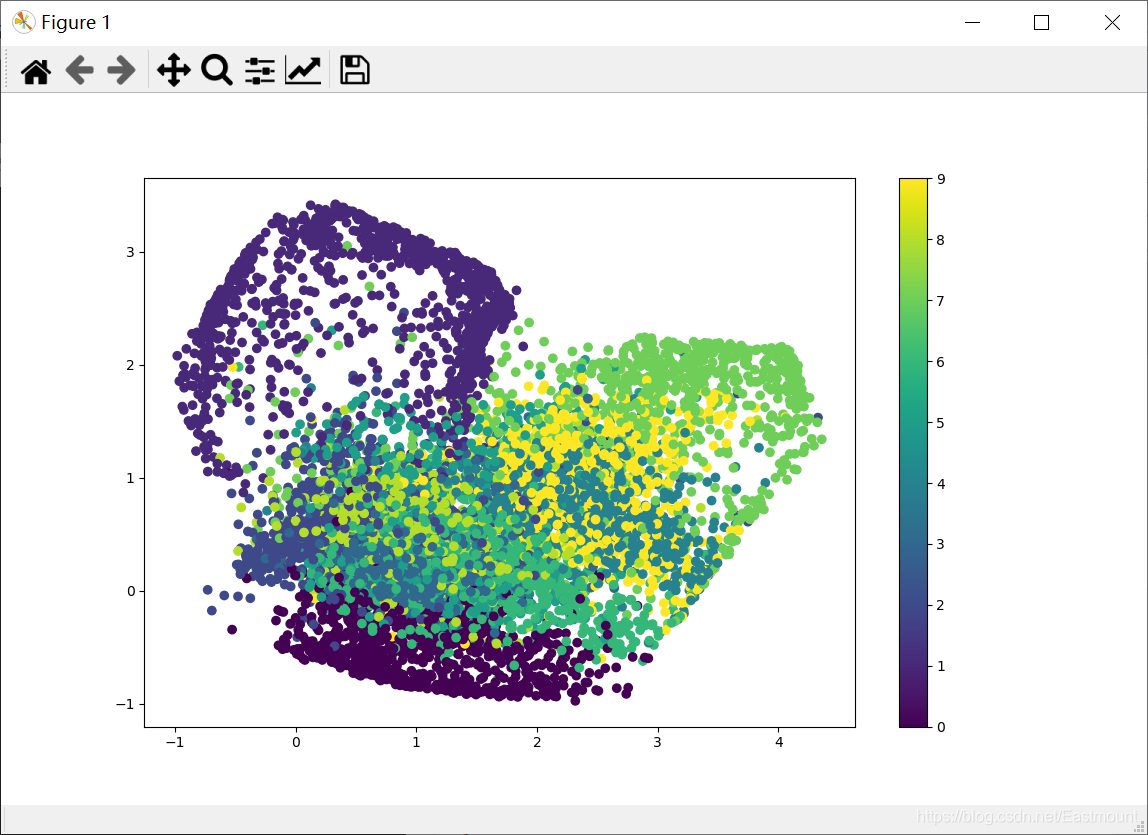# 一.什么是Autoencoder

(1) 首先，什么是自编码（Autoencoder）？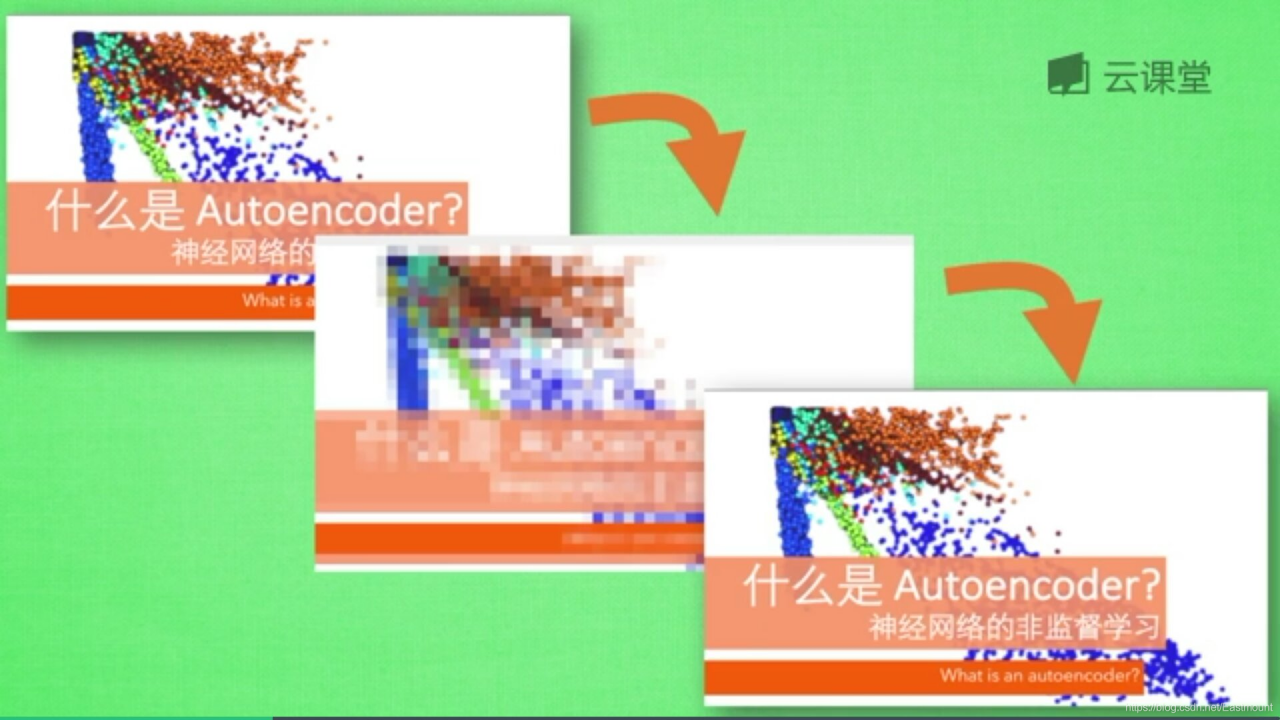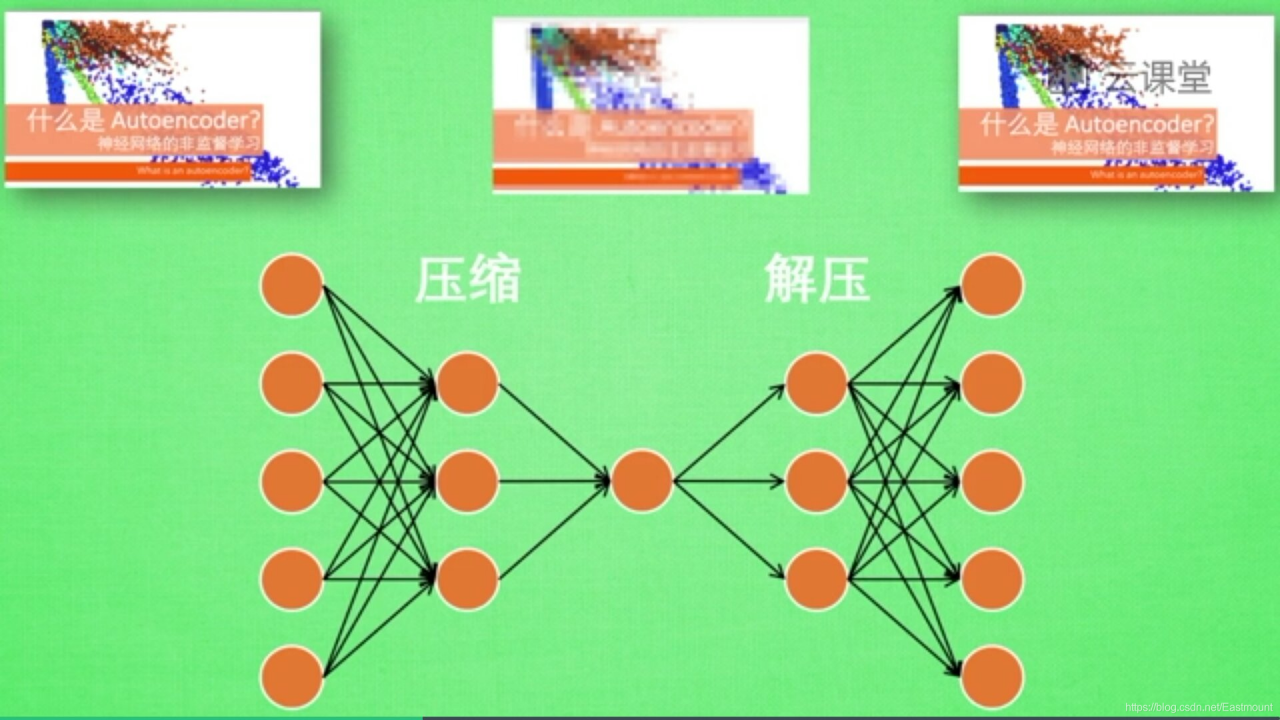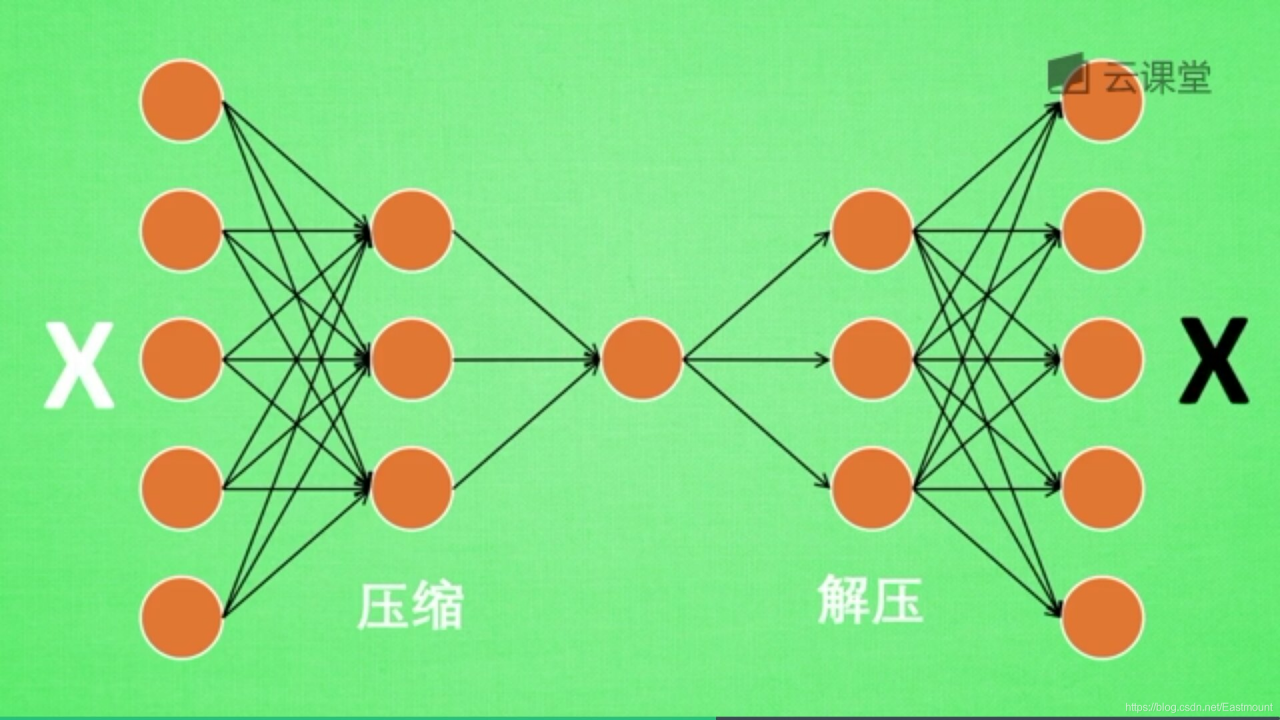(2) 自编码器的计算过程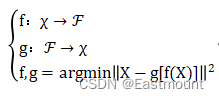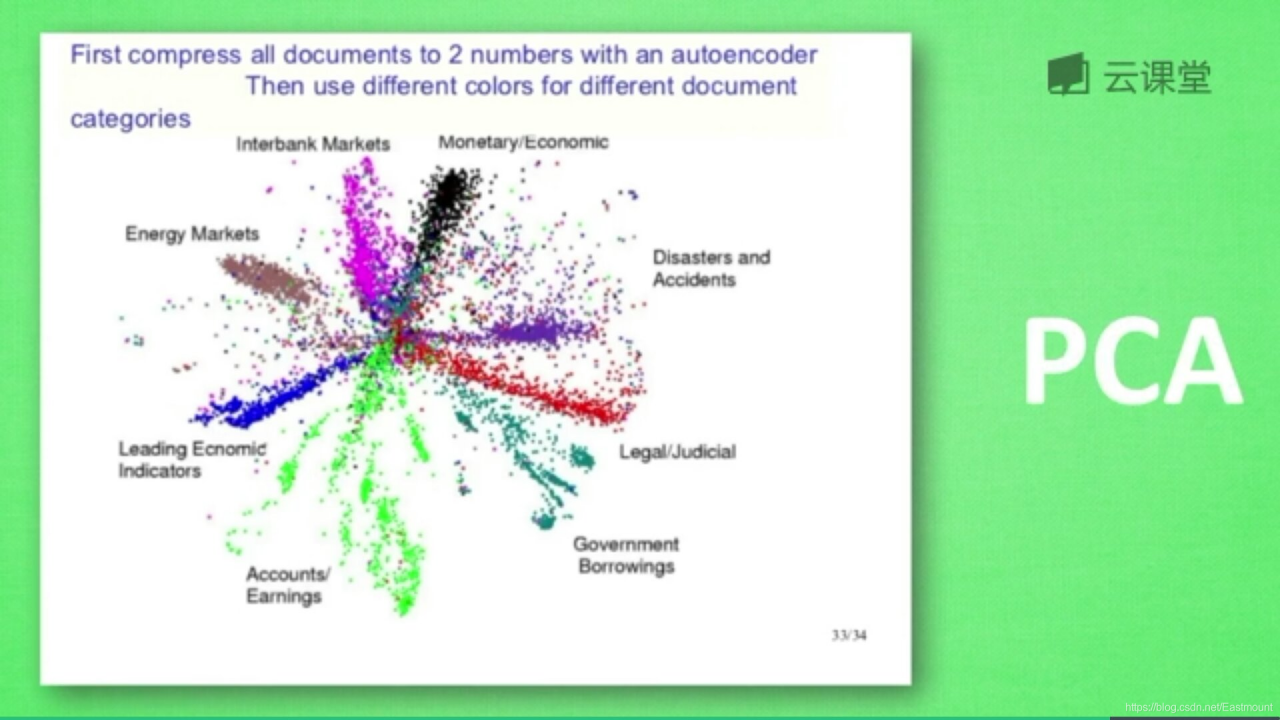(3) 自编码器的应用场景

• 降维（dimensionality reduction）
• 异常值检测（anomaly detection）
• 文本聚类
• 数据去燥
• 图像修复
• 信道压缩与重建
• 信息检索

——百度百科 https://baike.baidu.com/item/自编码器/23686966

# 二.Autoencoder分析MNIST数据

Autoencoder算法属于非监督学习，它是把数据特征压缩，再把压缩后的特征解压的过程，跟PCA降维压缩类似。本篇文章的代码包括两部分内容：

• 第一部分：使用MNIST数据集，通过feature的压缩和解压，对比解压后的图片和压缩之前的图片，看看是否一致，实验想要的效果是和图片压缩之前的差不多。
• 第二部分：输出encoder的结果，压缩至两个元素并可视化显示。在显示图片中，相同颜色表示同一类型图片，比如类型为1（数字1），类型为2（数字2）等等，最终实现无监督的聚类。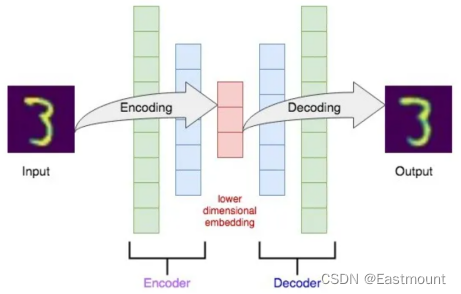(1) 有监督学习方法必须要有训练集与测试样本。在训练集中找规律，而对测试样本使用这种规律。而非监督学习没有训练集，只有一组数据，在该组数据集内寻找规律。
(2) 有监督学习的方法就是识别事物，识别的结果表现在给待识别数据加上了标签。因此训练样本集必须由带标签的样本组成。而非监督学习方法只有要分析的数据集的本身，预先没有什么标签。 如果发现数据集呈现某种聚集性，则可按自然的聚集性分类，但不予以某种预先分类标签对上号为目的。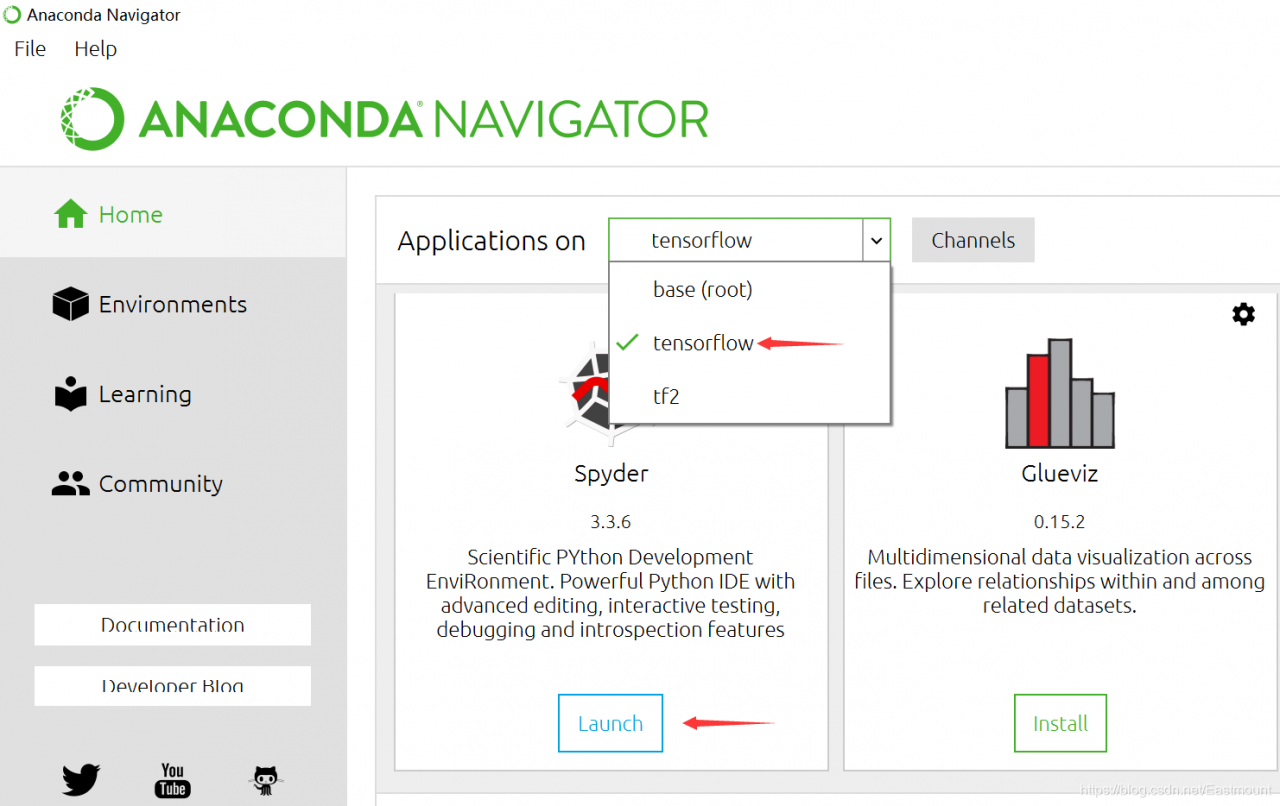``````import numpy as np
from keras.datasets import mnist
from keras.utils import np_utils
from keras.models import Model
from keras.layers import Dense, Input
import matplotlib.pyplot as plt
``````

• 注意，仅使用x数据集
``````#-------------------------下载MNIST数据--------------------------------
(x_train, _), (x_test, y_test) = mnist.load_data()
print(x_train.shape, x_test.shape, _.shape, y_test.shape)
``````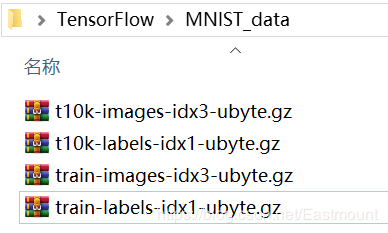``````(60000, 28, 28) (10000, 28, 28) (60000,) (10000,)
``````

``````#---------------------------数据预处理--------------------------------
x_train = x_train.astype('float32') / 255. - 0.5
x_test = x_test.astype('float32') / 255. - 0.5
x_train = x_train.reshape((x_train.shape, -1))
x_test = x_test.reshape((x_test.shape, -1))
print(x_train.shape, x_test.shape)
``````

``````(60000, 784) (10000, 784)
``````

``````#-----------------------构建Encoder和Decoder层-----------------------
#降维可视化绘制2D图
encoding_dim = 2

#input placeholder 28*28
input_img = Input(shape=(784,))

#Encoder layers（压缩）
encoded = Dense(128, activation='relu')(input_img)
encoded = Dense(64, activation='relu')(encoded)
encoded = Dense(10, activation='relu')(encoded)
encoder_output = Dense(encoding_dim,)(encoded)

#Decoder Layers（解压）
decoded = Dense(10, activation='relu')(encoder_output)
decoded = Dense(64, activation='relu')(decoded)
decoded = Dense(128, activation='relu')(decoded)
decoded = Dense(784, activation='tanh')(decoded)
``````

• encoded = Dense(128, activation=‘relu’)(input_img)
利用Dense构造Encoder层，其输出值为128，输入值为input_img
• encoded = Dense(64, activation=‘relu’)(encoded)
第二层的输出是64，输入是上一个构建的encoded
• encoded = Dense(10, activation=‘relu’)(encoded)
第三层压缩至10
• encoder_output = Dense(encoding_dim,)(encoded)
最后构建需要的自编码压缩器，压缩成2个值，它能代表整个784个特征，并且可用于聚类

• decoded = Dense(10, activation=‘relu’)(encoder_output)
• decoded = Dense(64, activation=‘relu’)(decoded)
• decoded = Dense(128, activation=‘relu’)(decoded)
• decoded = Dense(784, activation=‘tanh’)(decoded)
由于输入值是(-0.5,0.5)，而使用tanh激活函数的范围是(-1,1)，因此实现对应效果

``````#构造自编码器模型
autoencoder = Model(inputs=input_img, outputs=decoded)

#构建encoder模型进行可视化分析
encoder = Model(inputs=input_img, outputs=encoder_output)

#激活自编码器

``````

• 调用matplotlib库画图，可视化对比原始图像和预测图像
• 压缩结果聚类分析
``````#-----------------------------训练和测试------------------------------
#训练
autoencoder.fit(x_train,
x_train,
epochs=20,
batch_size=256,
shuffle=True)

#预测
encoded_imgs = encoder.predict(x_test)     #压缩二维特征 用于聚类
decoded_imgs = autoencoder.predict(x_test) #自编码器还原的图像

#比较原始图像和预测图像数据
f, a = plt.subplots(2, 10, figsize=(10, 2))
for i in range(10):
a[i].imshow(np.reshape(x_test[i], (28, 28)))
a[i].imshow(np.reshape(decoded_imgs[i], (28, 28)))
plt.show()

#聚类分析
plt.scatter(encoded_imgs[:,0], encoded_imgs[:,1], c=y_test)
plt.colorbar()
plt.show()
``````

``````(60000, 28, 28) (10000, 28, 28) (60000,) (10000,)
(60000, 784) (10000, 784)
Epoch 1/20
235/235 [==============================] - 2s 5ms/step - loss: 0.0697
Epoch 2/20
235/235 [==============================] - 1s 6ms/step - loss: 0.0564
Epoch 3/20
235/235 [==============================] - 1s 5ms/step - loss: 0.0516
Epoch 4/20
235/235 [==============================] - 1s 6ms/step - loss: 0.0493
Epoch 5/20
235/235 [==============================] - 1s 6ms/step - loss: 0.0475
Epoch 6/20
235/235 [==============================] - 1s 5ms/step - loss: 0.0462
Epoch 7/20
235/235 [==============================] - 1s 6ms/step - loss: 0.0453
Epoch 8/20
235/235 [==============================] - 1s 5ms/step - loss: 0.0446
Epoch 9/20
235/235 [==============================] - 1s 5ms/step - loss: 0.0439
Epoch 10/20
235/235 [==============================] - 2s 6ms/step - loss: 0.0432
Epoch 11/20
235/235 [==============================] - 2s 7ms/step - loss: 0.0427
Epoch 12/20
235/235 [==============================] - 2s 7ms/step - loss: 0.0421
Epoch 13/20
235/235 [==============================] - 2s 7ms/step - loss: 0.0418
Epoch 14/20
235/235 [==============================] - 2s 7ms/step - loss: 0.0414
Epoch 15/20
235/235 [==============================] - 2s 8ms/step - loss: 0.0411
Epoch 16/20
235/235 [==============================] - 2s 8ms/step - loss: 0.0409
Epoch 17/20
235/235 [==============================] - 2s 6ms/step - loss: 0.0406
Epoch 18/20
235/235 [==============================] - 2s 7ms/step - loss: 0.0404
Epoch 19/20
235/235 [==============================] - 2s 7ms/step - loss: 0.0401
Epoch 20/20
235/235 [==============================] - 2s 7ms/step - loss: 0.0400
``````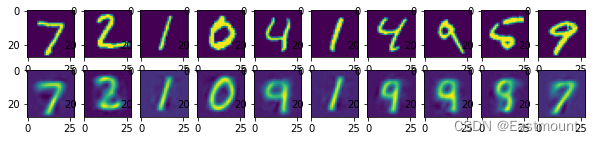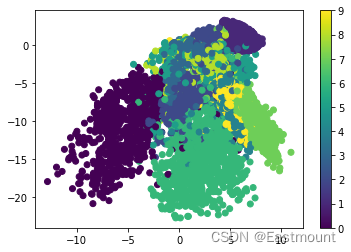# 三.完整代码

``````# -*- coding: utf-8 -*-
# By：Eastmount CSDN 2022-08-23
import numpy as np
from keras.datasets import mnist
from keras.utils import np_utils
from keras.models import Model
from keras.layers import Dense, Input
import matplotlib.pyplot as plt

#-------------------------下载MNIST数据--------------------------------
#只使用x数据集
(x_train, _), (x_test, y_test) = mnist.load_data()
print(x_train.shape, x_test.shape, _.shape, y_test.shape)

#---------------------------数据预处理--------------------------------
#minmax_normalized 处理至(-0.5,0.5)区间
x_train = x_train.astype('float32') / 255. - 0.5
x_test = x_test.astype('float32') / 255. - 0.5
x_train = x_train.reshape((x_train.shape, -1))
x_test = x_test.reshape((x_test.shape, -1))
print(x_train.shape, x_test.shape)

#-----------------------构建Encoder和Decoder层-----------------------
#降维可视化绘制2D图
encoding_dim = 2

#input placeholder 28*28
input_img = Input(shape=(784,))

#Encoder layers（压缩）
#利用Dense构造Encoder层，其输出值为128，输入值为input_img
encoded = Dense(128, activation='relu')(input_img)
#第二层的输出是64，输入是上一个构建的encoded
encoded = Dense(64, activation='relu')(encoded)
#第三层压缩至10
encoded = Dense(10, activation='relu')(encoded)
#最后构建需要的自编码压缩器，压缩成2个值，它能代表整个784个特征
encoder_output = Dense(encoding_dim,)(encoded)

#Decoder Layers（解压）
#通常Encoder怎么构建，Decoder也对应反向构建，实现解压处理，重构至784个特征
decoded = Dense(10, activation='relu')(encoder_output)
decoded = Dense(64, activation='relu')(decoded)
decoded = Dense(128, activation='relu')(decoded)
#由于输入值是(-0.5,0.5)，而使用tanh激活函数的范围是(-1,1)，因此实现对应效果
decoded = Dense(784, activation='tanh')(decoded)

#--------------------------构造自编码器模型---------------------------
autoencoder = Model(inputs=input_img, outputs=decoded)

#构建encoder模型进行可视化分析
encoder = Model(inputs=input_img, outputs=encoder_output)

#激活自编码器

#-----------------------------训练和测试------------------------------
#输入和输出均是x_train,对比二者形成误差
autoencoder.fit(x_train,
x_train,
epochs=20,
batch_size=256,
shuffle=True)

#预测
encoded_imgs = encoder.predict(x_test)     #压缩二维特征 用于聚类
decoded_imgs = autoencoder.predict(x_test) #自编码器还原的图像

#比较原始图像和预测图像数据
f, a = plt.subplots(2, 10, figsize=(10, 2))
for i in range(10):
a[i].imshow(np.reshape(x_test[i], (28, 28)))
a[i].imshow(np.reshape(decoded_imgs[i], (28, 28)))
plt.show()

#聚类分析
plt.scatter(encoded_imgs[:,0], encoded_imgs[:,1], c=y_test)
plt.colorbar()
plt.show()
``````

# 四.总结(By:Eastmount 2022-08-23 夜于武汉 http://blog.csdn.net/eastmount/ )

 杨秀璋, 颜娜. Python网络数据爬取及分析从入门到精通（分析篇）[M]. 北京：北京航天航空大学出版社, 2018.
 “莫烦大神” 网易云视频地址
 https://study.163.com/course/courseLearn.htm?courseId=1003209007
 https://github.com/siucaan/CNN_MNIST
 https://github.com/eastmountyxz/AI-for-TensorFlow
《机器学习》周志华
 深度学习（07）RNN-循环神经网络-02-Tensorflow中的实现 - 莫失莫忘Lawlite
 https://github.com/lawlite19/DeepLearning_Python